##### International Journal of
###### eISSN: 2576-4454# HydrologyReview Article Volume 3 Issue 6

# SCS-CN method revisited in perspective of strange data

#### Mishra SK, function clickButton(){ var name=document.getElementById('name').value; var descr=document.getElementById('descr').value; var unCopyslNo=document.getElementById('unCopyslNo').value; document.getElementById("mydiv").style.display = "none"; $.ajax({ type:"post", url:"https://medcraveonline.com/captchaCode/server_action", data: { 'name' :name, 'descr' :descr, 'unCopyslNo': unCopyslNo }, cache:false, success: function (html) { //alert('Data Send');$('#msg').html(html); } }); return false; } Verify Captcha Regret for the inconvenience: we are taking measures to prevent fraudulent form submissions by extractors and page crawlers. Please type the correct Captcha word to see email ID.function refreshPage(){ $("#mydiv").load(location.href + " #mydiv"); }$(document).ready(function () { //Disable cut copy paste $('#msg').bind('cut copy paste', function (e) { e.preventDefault(); }); //Disable mouse right click$("#msg").on("contextmenu",function(e){ return false; }); }); .noselect { -webkit-touch-callout: none; /* iOS Safari */ -webkit-user-select: none; /* Safari */ -khtml-user-select: none; /* Konqueror HTML */ -moz-user-select: none; /* Firefox */ -ms-user-select: none; /* Internet Explorer/Edge */ user-select: none; /* Non-prefixed version, currently supported by Chrome and Opera */ cursor: none; } Kumre SK, Ashish Pandey

Department of Water Resources Development & Management, Indian Institute of Technology Roorkee, India

Correspondence: Mishra SK, Department of Water Resources Development & Management, Indian Institute of Technology Roorkee, Roorkee-247 667, Uttarakhand, India

Received: July 20, 2017 | Published: December 19, 2019

Citation: Mishra SK, Kumre SK, Pandey A. SCS-CN method revisited in perspective of Strange data. Int J Hydro. 2019;3(6):488-498. DOI: 10.15406/ijh.2019.03.00215

# Abstract

This paper revisits the popular rainfall-runoff Soil Conservation Service Curve Number (SCS-CN) methodology in perspective of Strange (1892),1 rainfall-runoff procedure widely used in Southern parts of India. It amends the Hawkins’ (1993) concept of CN decaying with increasing rainfall (P) for complacent watersheds, which contrasts the general notion that the runoff coefficient (C) (or CN, another form of C) increases with increasing P. Its roots lie in the existing potential maximum retention (S)-CN mapping relationship. Besides suggesting a rational modification to this relationship, a more rational form of the SCS-CN methodology is presented and is found to work satisfactorily on the Strange data.

Keywords: complacent watershed, curve number, CN-P relationship, NEH-4, rainfall-runoff relation, Soil Conservation Service Curve Number method, Strange table

# Introduction

The Soil Conservation Service Curve Number (SCS-CN) method2,3 is popularly used for estimation of direct runoff for a given rainfall event from small agricultural watersheds. Most of the commercial watershed models such as CREAMS,4 AGNPS,5 EPIC,6 and SWAT7 employ this methodology because of its simplicity, ease of use, lesser input data requirements, use of major runoff producing characteristics, widespread acceptance, and significant infrastructure and institutional momentum for this procedure within Natural Resource Conservation Service (NRCS).8

The conceptual/empirical SCS-CN model of hydrologic abstraction requires basic descriptive inputs smoothly converted into numeric values of CN9 reflecting the runoff potential of the watershed.10 The method takes into account the major runoff producing watershed characteristics, such as soil type, land use/ treatment, surface condition, and antecedent moisture conditions (AMCs). Since its inception, the method has been applied in several areas other than originally intended, as for example long-term hydrologic simulation,4,11‒23 prediction of infiltration & rainfall-excess rates and hydrograph simulation,24 sediment yield modeling,25-29 partitioning of heavy metals,30,31 determination of subsurface flow,32 urban hydrology and rainwater harvesting,33,34 water quality,35 and distributed hydrologic modeling25,36,37 using GIS and remote sensing. For determination of subsurface flow, Yuan et al.32 modified the SCS-CN technique based on analogy derived from the plot of accumulated subsurface drainage flow against accumulated infiltration yielding subsurface drainage flow to have started after some infiltration had accumulated and the relationship to have become asymptotic to a line of 45° slope, quite similar to the popular SCS-CN rainfall-runoff relationship. The procedure was testd using the data of Little Vermilion River (LVR) watershed in East-Central Illinois.

Strange (1892)1 studied the available rainfall and runoff in border areas of the present-day Maharashtra and Karnataka States of India and obtained runoff to rainfall ratios as functions of indicators representing catchment characteristics. These catchments were classified as Good, Average, and Bad according to their relative magnitudes of runoff coefficient. Table 1 shows the runoff coefficients (%) for different monsoon rainfall values. Here, it is presumed that the SCS-CN concept is applicable to rainfall-runoff data of any duration derived from a drainage area irrespective of its size.

 Total monsoon rainfall (mm) Runoff coefficient (%) Total monsoon rainfall (mm) Runoff coefficient (%) Good catchment Average catchment Bad catchment Good catchment Average catchment Bad catchment 1 2 3 4 1 2 3 4 25.4 0.1 0.1 0.1 787.4 27.4 20.5 13.7 50.8 0.2 0.2 0.1 812.8 28.5 21.3 14.2 76.2 0.4 0.3 0.2 838.2 29.6 22.2 14.8 101.6 0.7 0.5 0.3 863.6 30.8 23.1 15.4 127 1 0.7 0.5 889 31.9 23.9 15.9 152.4 1.5 1.1 0.7 914.4 33 24.7 16.5 177.8 2.1 1.5 1 939.8 34.1 25.5 17 203.2 2.8 2.1 1.4 965.2 35.3 26.4 17.6 228.6 3.5 2.6 1.7 990.6 36.4 27.3 18.2 254 4.3 3.2 2.1 1016 37.5 28.1 18.7 279.4 5.2 3.9 2.6 1041.4 38.6 28.9 19.3 304.8 6.2 4.6 3.1 1066.8 39.8 29.8 19.9 330.2 7.2 5.4 3.6 1092.2 40.9 30.6 20.4 355.6 8.3 6.2 4.1 1117.6 42 31.5 21 381 9.4 7 4.7 1143 43.1 32.3 21.5 406.4 10.5 7.8 5.2 1168.4 44.3 33.2 22.1 431.8 11.6 8.7 5.8 1193.8 45.4 34 22.7 457.2 12.8 9.6 6.4 1219.2 46.5 34.8 23.2 482.6 13.9 10.4 6.9 1244.6 47.6 35.7 23.8 508 15 11.3 7.5 1270 48.8 36.6 24.4 533.4 16.1 12 8 1295.4 49.9 37.4 24.9 558.8 17.3 12.9 8.6 1320.8 51 38.2 25.5 584.2 18.4 13.8 9.2 1346.2 52.1 39 26 609.6 19.5 14.6 9.7 1371.6 53.3 39.9 26.6 635 20.6 15.4 10.3 1397 54.4 40.8 27.2 660.4 21.8 16.3 10.9 1422.4 55.5 41.6 27.7 685.8 22.9 17.1 11.4 1447.8 56.6 42.4 28.3 711.2 24 18 12 1473.2 57.8 43.3 28.9 736.6 25.1 18.8 12.5 1498.6 58.9 44.4 29.4 762 26.3 19.7 13.1 1524 60 45 30

Table 1 Strange Table of total Monsoon rainfall and the percent runoff coefficients1

As seen from Table 1, the runoff coefficient (C) (Col. 2) (ratio of direct surface runoff, Q, to rainfall, P (Col. 1)), increases with increasing P, and vice versa. Since, from SCS-CN concept.10 CN increases as C increases for a given amount of rainfall, CN should increase with P as does C. However, while describing the behavior of watersheds as complacent or standard, CN is shown to decrease with P whereas violent watersheds exhibit a decrease in CN with P up to a certain extent and afterwards, CN increases with P sharply.38 To circumvent the problem, a modification is proposed to the proportional equality of the SCS-CN methodology10 and the S-CN mapping relationship. Thus, the primary objective of this paper is to resolve this issue and evaluate the proposed models using Strange data.

# Methodology

Existing SCS-CN methodology

The SCS-CN method is based on the water balance equation and two hypotheses expressed, respectively, as:

$P={I}_{a}+F+Q$ (1a)

$\frac{Q}{\left(P-Ia\right)}=\frac{F}{S}$ (1b)

$Ia=\lambda S$                   (1c)

Where P, total precipitation; Ia, initial abstraction; F, cumulative infiltration excluding Ia; Q, direct runoff; and S, potential maximum retention or infiltration. The current version of the SCS-CN method assumes coefficient of initial abstraction (λ) equal to 0.2 in routine applications. λ can range from 0 to ∞.10 Combining Eqs. (4) and (5) leads to

$Q=\frac{{\left(P-Ia\right)}^{2}}{P-Ia+S}$ (2a)

λ = 0.2, $Q=\frac{{\left(P-0.2S\right)}^{2}}{P+0.8S}$        (2b)

Eq. (2) is valid for P≥Ia; Q=0 otherwise. Eq. 2a is the general form (designated as Model 1) of Eq. 2b (designated as Model 2), which is the popular form of the existing SCS-CN methodology. The only parameter S can range 0≤S≤∞, and therefore, it is mapped onto a dimensionless curve number CN, varying from 0≤CN≤100, as:

$S=\frac{25400}{CN}-254$                   (3)

Where S is in mm. CN=100 represents a condition of zero potential maximum retention (S=0), that is a completely non-abstracting impermeable watershed. On the other hand, CN=0 represents (S=∞), that is an infinitely abstracting watershed. Mishra and Singh10 described CN as an index of runoff potential of a watershed corresponding to 10 inches (=254mm) of rainfall. This logic is also supported by the fact that routine RS-GIS applications frequently distinguish two watersheds in terms of CN for their runoff-producing (or hydrologic) potential, ignoring the fact that CN also depends on rainfall. Thus, it is more rational to describe the runoff producing potential of a watershed for a particular rainfall amount.

S can be determined from Eq. 2b as:

$S=5\left(P+2Q-\sqrt{\left(Q\left(4Q+5P\right)}\right)$ (4)

Notably, the SCS-CN method excludes the effect of slope on runoff yield and, in turn, on the resulting CN.

Significance of l

l (Eq. 1c) actually represents the ratio of initial abstraction (Ia) to parameter S. Since Ia included climate-dependent evaporation, soil-dependent initial infiltration, surface feature (land use)-dependent surface detention, and vegetal (land cover) interception, and all these affect the surface runoff potential described by CN (or S), and therefore, it is not out of order to describe it as a function (or fraction or multiple) of S (or CN). S, in turn, is mapped on to CN, and therefore, it is not out of order to foresee the dependency of l on CN (or C) that varies with P.38 Because of larger variability, Ia=0.2S relationship has been the focus of discussion in literature and modification since its inception. Aron et al.39 suggested l≤0.1 and Golding40 provided l values for urban watersheds depending on CN as l=0.075 for CN≤70, l=0.1 for 70<CN≤80, and l=0.15 for 80<CN≤90. Ponce & Hawkins41 suggested that the fixing of l as 0.2 might not be the most appropriate number, and that it should be interpreted as a regional parameter. Hawkins et al.42 found that a value of l=0.05 fitted the data better and therefore was more appropriate for use in runoff calculations.

Mishra & Singh43 suggested that λ can take any non-negative value. Mishra & Singh24 developed criterion for the applicability of SCS-CN method based on runoff coefficient (C) and l variation. They defined the applicability bounds for the SCS-CN method as for l≤0.3, Ia/P≤0.35 and C≥0.23. Since P relies on climate/meteorological characteristics of the region, Jain et al.17 proposed a more general non-linear Ia-S-P relation, and Mishra et al.44 used Ia-S-M relationship based on the hypothesis that Ia largely depends on the initial soil moisture (M). Thus, there exists a sufficient scope for improvement.

Significance of CN

Mishra and Singh10 described the significance of CN as a degree of saturation (Sr) of an initially completely dry watershed due to 254mm (=10inches) of rainfall. For Ia=0, Eq. 1a can be recast as,

$100\frac{F}{S}=C{N}_{P}=\frac{100}{1+S/P}$                     (5)

Which describes the P-dependent CNP for a given S. For P=254mm (=10inches), CNp=CN as described by Eq. 3. It is worth noting that the direct use of CN in the proportionality hypothesis (Eq. 1b) for computing Q is restricted, because CN, by definition, corresponds to the 254mm base rainfall amount, not to the actual amount. Therefore, Eq. 2 with Ia=0 should be resorted to computation of Q for a given rainfall amount.

In terms of CN, the runoff factor C (=Q/P) can be defined from Eq. 2 (for Ia=0) as:

$C=\frac{1}{1+\frac{254}{P}\left(\frac{100}{CN}-1\right)}$         (6)

Figure 1 depicting the variation of C with CN and P shows that for a given P, as C increases, CN also increases; for a given C, CN increases with decreasing P; and for a given CN, C increases as P increases. The first condition is realizable when the watershed characteristics change, as for example, from agriculture to urban or vice versa. The last condition of a given CN, implying a particular type of watershed with certain wetness or of specific runoff potential, is realizable as C increases with P, as shown by Strange data described later. However, the second condition is hard to realize for the reason that C is forced to remain constant with changing P, which it actually can’t, and therefore, CN (which is a constant) is forced to exhibit a decreasing trend with increasing P for enabling C to remain at a fixed value in Eq. 6. Such behaviour can also be explained as follows.Figure 1 Variation of runoff factor (C) with curve number (CN) and precipitation (P, inch). 1inch=25.4mm.

Re-writing Eq. 4 in terms of C and P, it can be shown that S (or SP) is a function of both C and P. C increases with P1 and S (or SP) is directly proportional to P (Eq. 4). Thus, S (or SP) will increase with increasing P, and vice versa, and, from Eq. 3, CN is inversely related to S (or SP). It leads to the inference that CN is inversely related to P. Such unrealistic behaviour has led to several misunderstandings/misinterpretations. To circumvent the problem, a modification is needed in the SCS-CN application procedure, as follows. From given P-Q data, compute SP from Eq. 4, and CNP from Eq. 5. Here, subscript ‘P’ to S or CN refers to their correspondence with P. Similarly, CN corresponding to 254mm of P can be defined as CN10 or simply CN from Eq. 3. Since it represents the runoff potential of a watershed for a fixed amount of rainfall, it is a better indicator to predict the comparative effect of watershed characteristics.

# Description of watershed behaviour

Following Hawkins,38 Strange data (Table 1) is plotted in Figure 2. In this figure, solid lines show the variation of CN (derived from Eqs. 4 and 3) with P for varying C (=Q/P) values. As seen, for any fixed C-value, CN decreases with P, as described above. The dotted lines correspond to three watersheds described by Strange as Good, Average, and bad watersheds depending on their runoff generating potential. A Good watershed exhibits a high and Bad a low runoff generating potential, and Average falls in between. Thus, consistent with the literature, let the Bad watershed be described as Complacent, Average as Standard, and Good as violent watershed. Notably, for a watershed to be violent, CN should increase rapidly with increasing P.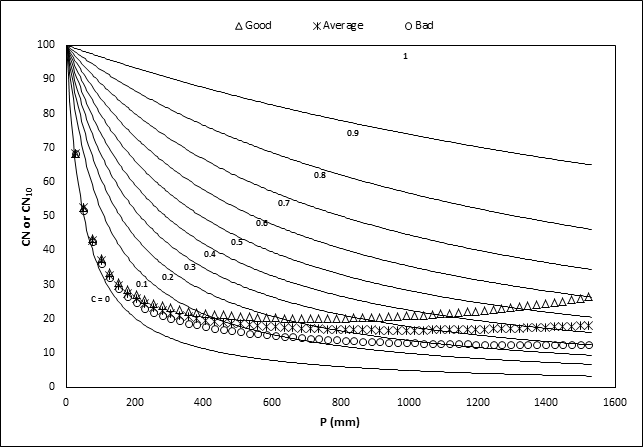Figure 2 Plot of Strange data in the existing CN perspective.

As seen from Figure 2, the complacent type behaviour of the watershed closely follows the line C=0. C can however be equal to 0 under three situations: (a) P£0.2S, (b) P=0, and (c) S=¥. Here, the second situation is clearly improbable for an event to occur (as P is non-negative) whereas the first and last ones are most likely to prevail. Thus, for C=0, P has always to be less than 0.2S, and therefore, has to follow C=0 line. In other words, complacent behaviour is realizable only if it is an absolutely zero runoff potential watershed, an idealized situation. On the other hand, CN values are seen in the same figure to range (12.63, 68.23). Similarly, the data of the standard watershed exhibits C to vary from 0.001 to 0.45 and CN values range (16.63, 68.23). The data of Good, Average, and Bad watersheds exhibit C to vary in the range (0.001, 0.6), (0.001, 0.45), and (0.001, 0.3), respectively. Up to the reasonably high rainfall of 254mm (=10inches), C-values range (0.001, 0.043), (0.001, 0.032), and (0.001, 0.021), respectively, indicating all watersheds to be low runoff producing watersheds.

Following the present understanding on the existing S-CN relationship (Eq. 3), the behaviour of the complacent watersheds can be described as follows. All three watersheds closely follow C=0 line until P exceeds Ia. It will exist under all circumstances in nature as the direct surface runoff always starts with zero, and therefore, every watershed has to follow it and, in turn, has to be complacent in nature. When P exceeds Ia, the behaviour of watershed is actually reflected by the increase in both P and P-dependent C, rather than CN (which is for P=254mm). The gradual and abrupt rate of rise in C leads to the description of watersheds as standard and violent, respectively. At what P-magnitude, this rise will be experienced in a watershed will depend on watershed characteristics affecting the runoff generating potential.

Figure 3 shows CN-P and CNP-P relations to describe the behaviour of three types of watersheds described by Strange. As seen, CNP exhibits a more rational behaviour than does CN with increasing P for all three datasets; as P increases, CNP also increases and C (not shown) also increases, consistent with the above notion.Figure 3 CN and CNP versus P relations for strange datasets. Note: Strange data best fitted with CN using 2 period moving averages.

The methodology proposed (designated as Model 3 for CN and Model 4 for CNp) can be described in steps as follows:

1. Determine S or SP from Eq. 4 using the observed P-Q dataset for a watershed.
2. Determine CN from Eq. 3 and CNP from Eq. 5.
3. Develop a relationship between CN or CNP and P for the watershed.
4. For known P, derive CN or CNP from the respective relation for field application.

Application of these models is demonstrated using Strange (1892) data.

Modification to the formulation of SCS-CN methodology

The basic formulation of SCS-CN methodology is modified keeping in view the decreasing trend of CN with P in Figure 3. To this end, the proportional equality hypothesis is re-written and revised as follows:

$\frac{F\left(P-{I}_{a}\right)}{Q\text{\hspace{0.17em}}}=S={S}_{o}{e}^{-\alpha P}$                                      (7)

Where So is the initial storage space (or potential maximum retention when P=0) (mm) and α is a decay coefficient (mm-1). The right hand side of Eq. 7 is consistent with the work of Mishra and Singh,10 the storage space actually decreases as rainfall grows with time. Taking Ia=0, Eq. 7 can be reformulated as:

$Q=\frac{{P}^{2}}{P+\text{\hspace{0.17em}}{S}_{o}{e}^{-\alpha P}}$                                    (8)

Which is the revised model formulation. From Eq. 8,

$\frac{{S}_{o}}{P}=\frac{1-C}{C\text{\hspace{0.17em}}{e}^{-\alpha P}}$                         (9)

Following Eq. 5, revised CN can be described as follows:

$C{N}_{o}=\frac{100}{1+\text{\hspace{0.17em}}{S}_{o}/P}=\frac{100\text{\hspace{0.17em}}C\text{\hspace{0.17em}}{e}^{-\alpha P}}{1+\left({e}^{-\alpha P}-1\right)C}$    (10)

For CNo0, C≥0 and/or P£¥, which is justifiable. For CNo£100, C£1, which is again reasonable. However, Eq. 10 shows CN to decrease with P, but for constant C, a physically unrealizable condition. When C=0, CNo=0 and when C=1, CNo=100. In addition, α=0 in Eq. 8 implies that So does not vary with precipitation (or time),10 which contrasts the fundamental decay behaviour of infiltration with time. Thus, Eq. 8 presents a more realistic formulation than the existing one. It is designated as Model 5 in the forthcoming text.

Model 5 (Eq. 8) can be further (coarsely) approximated to

$Q=\frac{{P}^{2}}{P+\text{\hspace{0.17em}}{S}_{o}\left(1-\alpha P\right)}$                                                                                                                   (11)

Or                                       $Q=\frac{{P}^{2}}{\left(1-\alpha {S}_{o}\right)P+\text{\hspace{0.17em}}{S}_{o}}=\frac{{P}^{2}}{aP+\text{\hspace{0.17em}}{S}_{o}}$                (12)

Where a=1– αSo. Eq. 12 is the Modified SCS-CN model proposed by Mishra and Singh (1999). From Eq. 12,

$\frac{{S}_{o}}{P}=\frac{1-C}{\left(1-\alpha P\right)C}$                                                                   (13)

As C®0, So/P® ¥, and as C®1, So/P® 0. From Eq. 5,

$CN=\frac{100\left(1-\alpha P\right)C}{1-\alpha PC}$                (14)

As C®0, CN®0, and as C®1, CN®100, consistent with the general notion that CN increases with C, and vice versa. Similar to Model 5, CN can be shown to decrease with P, but for constant C, a physically unrealizable condition. Furthermore, for CN to be non negative (the applicability criterion),

(15)

Eq. 11 or Eq. 12 is designated as Model 6 in the forthcoming text.

# Application

As above, Strange described the watersheds as Good, Average, and Bad according to their relative magnitudes of yield or runoff. It is again worth emphasizing here that the Strange monsoon season data (Table 1) was used considering that the SCS-CN concept is applicable to any duration (including seasonal) rainfalls. The correlation equations of best fitting lines relating percentage C are expressed as Strange (1892):

For Good catchment:

(16a)

(16b)

(16c)

For Average catchment:

(17a)

(17b)

(17c)

(18a)

(18b)

(18c)

Where C(%) is percentage; runoff coefficient, ratio of seasonal runoff to seasonal rainfall in percent (non-dimensional), P, monsoon season rainfall in mm, and R2, coefficient of determination.

Since there is no appreciable runoff due to rains in dry (non-monsoon) period, the monsoon season runoff volume has been taken as annual yield of the catchment.1 This table can be used to estimate the monthly yields also in the monsoon season. It is however to be used with the understanding that the table relates cumulative monthly rainfall since the beginning of the season and the corresponding cumulative runoff.

Considering the above Strange data as observed, the use of both CN and CNP concepts is shown to describe this data in Figure 3. As seen, CN first decreases with increase in P and then after a certain extent (i.e. P=254mm =10inches), CN increases with increasing P. Thus, the same CN-concept shows two different types of behaviour with increasing P. It is resolved by plotting CNP against P (Figure 3). This data has been used for performance evaluation of 6models described above and summarized in Table 2.

 Model No. Equations Parameter(s) Procedure 1 2a, 3 λ, S or CN from eq. 3 Optimize parameters 2 2b, 3 S (or CN from Eq. 3) Optimize parameters 3 2b, 3, 4 CN a) Determine S from Eq. 4 for each P-Q dataset for a watershed. (for P = 254mm) b) Determine CN from Eq. 3. c) Develop a relationship between CN and P for the watershed (Fig. 4) for future applications. d) Derive CN from P, then S from Eq. 3, and then Q from Eq. 2b. 4 2b, 4, 5 CNp a) Determine Sp from Eq. 4 for each P-Q dataset for a watershed. (for P = 254mm) b) Determine CNp from Eq. 5 or 10. c) Develop a relationship between CNp and P for the watershed (Fig. 4) for future applications. d) Derive CNp from P, then Sp from Eq. 5 or 10, and then Q from Eq. 2b. 5 8, 10 α, So (or CNo from Eq. 3) Optimize parameters 6 11, 14 α, So (or CNo from Eq. 3) Optimize parameters

Table 2 Model formulations/procedures

The model application results are shown in Table 3 and Figures 4A-4C. As seen, Model 1 is the generalized form of the existing SCS-CN model, i.e. Model 2. CN for both the models is derived from Eq. 3. Both Models 3 & 4 are based on Eq. 2b and Eq. 4. The former employs Eq. 3 for CN determination whereas the latter one employs Eq. 5 for CNp determination. CN- and CNp-values for these models are derived from the following relations (Figure 3):

 Sl. No. Watershed Type No. of P-Q events Mean rainfall (mm) C=Mean runoff/Mean rainfall Model 1 Model 2 λ S (CN) (mm) Eff. (%) Bias (mm) S (CN) (mm) Eff. (%) Bias (mm) 1 Good 60 774.7 0.38 0.29 746.42 98.88 6.34 869.49 98.69 6.73 25.39 22.61 2 Average 60 774.7 0.28 0.17 1311.94 99.61 1.84 1240.12 99.49 -2.39 16.22 17.00 3 Bad 60 774.7 0.19 0.07 2666.84 99.92 -0.03 1737.76 98.11 -0.26 8.69 12.75
 Sl. no. Model 3 Model 4 CN (for P=254mm) Eff. (%) Bias (mm) CNP (for P=254mm) Eff. (%) Bias (mm) 1 30.66 94.9 -40.05 25.15 99.98 -0.27 2 25.48 96.98 -8.78 23.84 99.95 0.05 3 23.09 99.82 -4.49 22.37 99.82 0.46
 Sl. No. Model 5 Model 6 α (mm-1) So (CNo) (mm) Eff. (%) Bias (mm) α (mm) So (CNo) (mm) Eff. (%) Bias (mm) 1 0.00099 4620.69 99.99 0.89 0.00045 3197.53 99.94 3.02 (5.21) (7.36) 2 0.00069 5300.71 98.98 1.18 0.00038 4294.18 99.94 2.17 (4.57) (5.58) 3 0.00047 7176.06 99.97 1.1 0.00029 6430.47 99.95 1.46 (3.42) (3.79)

Table 3 Performance evaluation of various models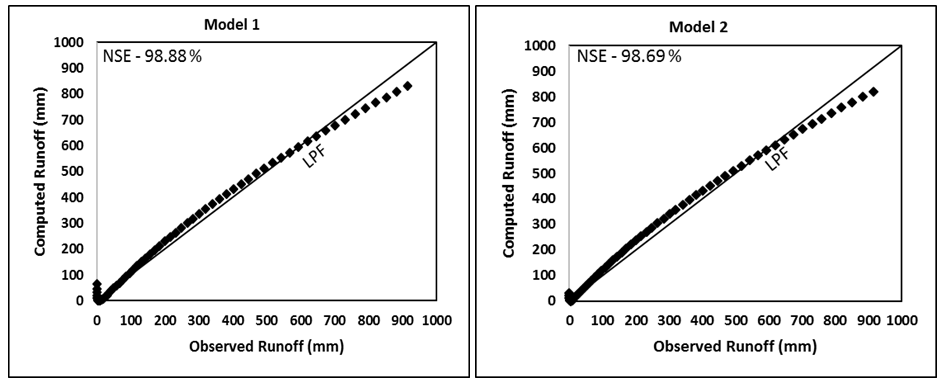Figure 4A Evaluation of Models 1-6 by comparing the computed runoff (mm) with the observed runoff (mm) of Good watershed (Strange, 1892). LPF represents the line of perfect fit. NSE, Nash and Sutcliffe Efficiency.Figure 4B Evaluation of Models 1-6 by comparing the computed runoff (mm) with the observed runoff (mm) of Average watershed (Strange, 1892). LPF represents the line of perfect fit. NSE, Nash and Sutcliffe Efficiency.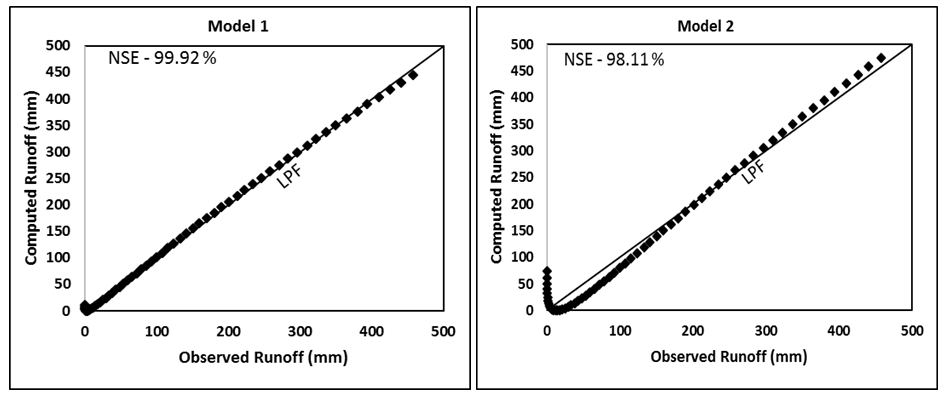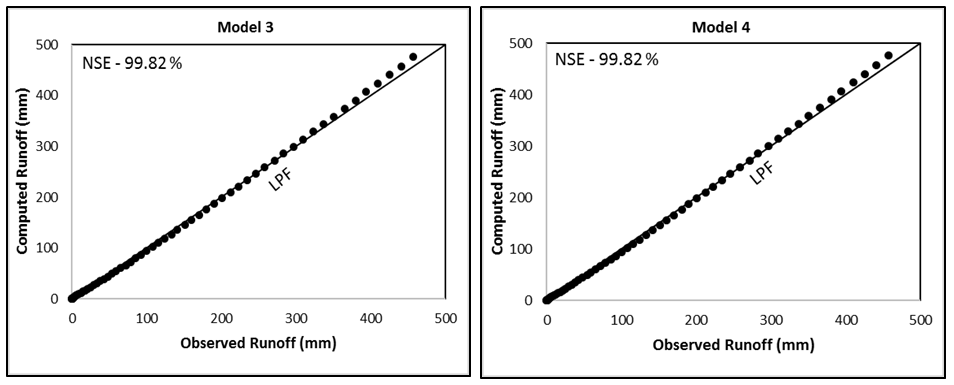Figure 4C Evaluation of Models 1-6 by comparing the computed runoff (mm) with the observed runoff (mm) of Bad watershed (Strange, 1892). LPF represents the line of perfect fit. NSE, Nash and Sutcliffe Efficiency.

For Model 3,

CN=3E-06P-0.0571P+43.225           (for Good watershed)                                                          (19a)

CN=134.89P-0.301                                  (for Average watershed)                                     (19b)

These CN relations can be further improved/simplified significantly by using CNP in place of CN (for Model 4),

CNp=0.0343P+16.435                       (for Good watershed)                                                          (20a)

CNp=0.0267P+17.06                          (for Average watershed)                                     (20b)

In Eqs. 10 & 14, 0£CN or CNp£100. Model 5 is based on Eq. 8, and Model 6 on Eq. 11. The former is the general form of the latter. CNo for both these models is derived from Eq. 3 so as to bring all CN-values at one P (=254mm = 10inch)-scale.

As seen from Table 3, Good watershed has the highest runoff coefficient (C) (derived from mean values of rainfall and runoff), Bad the lowest, and Average in between these two. Such order of runoff generating potential is described by CN (or CNo) values derived (for the same P=254mm) from all model applications. All the models generally performed extremely well on all watersheds. Model 3 performed the poorest. l of Model 1 is seen to have ranged from 0.07 (for Bad watershed) to 0.29 (for Good watershed). Thus, l appears to be mean C (or CN)-dependent. It is of paramount importance in field applications as a proper prescription of average C-dependent l-value can enhance the results significantly. l®0 appears to be reasonable for Bad type of watersheds, largely for the reason that such watersheds exhibit very high S (or low CN) value to describe a certain value of initial abstraction (Ia=lS). Similarly, a relatively high l-value is proper for Good type of watersheds as these watersheds exhibit very low S (or very high CN) values. Bias in Table 3 is presented to indicate whether a model over (positive)- or under (negative)-predicted the runoff.

The application results of Models 3 and 4 indicate that the latter is in general an improvement over the former one and, therefore, assert that the pre-derived CNp-P relationship for a watershed can be an improved alternative for runoff predictions using Model 2. In addition, as shown in Figure 5, the use of Eq. 5 better describes the Good, Average, and Bad watersheds just based on CNp or C-values.Figure 5 C-CNp relationship (Model-4 consisting of Eqs. 2b, 4, and 6) for description of watershed behaviour.

Model 5 is a general form of Model 6, and it is also exhibited by their application results. Both the parameters α and So, respectively, exhibit consistently decreasing and increasing trends with Good to Average and to Bad watersheds. In addition, Eq. 8 of Model 5 when plotted for a specific value of α, the resulting C-CNo (Figure 6) and CNo-P (Figure 7) relations more rationally describe the behavior of the three types of watersheds.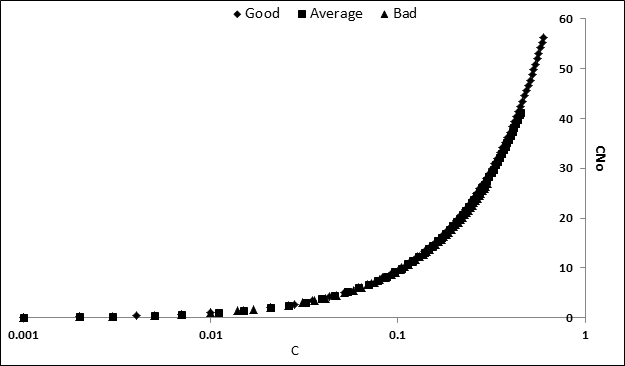Figure 6 C-CNo relationship for Model 5 (α=0.0001).Figure 7 Variation of CN with P (mm) for three types of watersheds for Model 5 with α=0.0001.

Thus, in order of preference all the models can be preferred for generally all watersheds. Among these, Model 3 is the least preferable. For improved applications, mean C-l can be prescribed for a watershed. Model 4 can be preferred if CNp-P relations are established. Model 6 along with mean C-α relation can also be a substitute for all watersheds.

# Conclusions

The following can be derived from the present study:

1. The description of three Good, Average, and Bad watersheds based on decreasing CN trend with increasing P is physically not justifiable as it contrasts the increasing trend of C (or CN) with increasing P.
2. The proposed CNp-P relation (Eq. 5) describes more rationally the behaviour of the above three watersheds.
3. The proposed modification to the application approach of the popular SCS-CN methodology (i.e. Model 4) is more rational, as it describes consistent C-CN-P behavior, and has the efficacy to describe the watershed behavior more scientifically and resolve the issue of CN decaying with increasing rainfall (P).
4. The proposed Models 5 & 6 have the power to physically describe the behavior of three watersheds and are equally suitable for these watersheds.
5. Model 1 and Model 2 performed extremely well for all the watersheds. However, the prescription of mean C-l (Model 1) and/or CNp-P (Model 4) relationships for a watershed can improve the application results significantly.45‒47

None.

# Conflicts of interest

The authors declare that there is no conflict of interest.

None.

# References©2019 Mishra, et al. This is an open access article distributed under the terms of the, which permits unrestricted use, distribution, and build upon your work non-commercially.